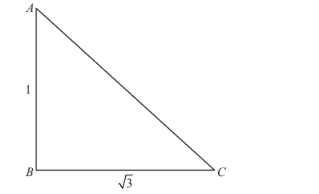# Mark the correct alternative in each of the following:

Question:

Mark the correct alternative in each of the following:

The ratio of the length of a rod and its shadow is $1: \sqrt{3}$. The angle of elevation of the sum is

3"> The angle of elevation of the sum is
(a) 30°

(b) 45°

(c) 60°

(d) 90°

Solution:

Letbe angle of elevation of sun.Given that: length of roadand its shadowHere, we have to find angle of elevation of sun.

So we use trigonometric ratios.

In a triangle,

$\Rightarrow \tan C=\frac{A B}{B C}$

$\Rightarrow \tan \theta=\frac{1}{\sqrt{3}}$  $\left[\because \tan 30^{\circ}=\frac{1}{\sqrt{3}}\right]$

$\Rightarrow \theta=30^{\circ}$

Hence the correct option is $a$.